## Selina Concise Mathematics Class 9 ICSE Solutions Compound Interest (Using Formula)

APlusTopper.com provides step by step solutions for Selina Concise Mathematics Class 9 ICSE Solutions Chapter 3 Compound Interest (Using Formula). You can download the Selina Concise Mathematics ICSE Solutions for Class 9 with Free PDF download option. Selina Publishers Concise Mathematics for Class 9 ICSE Solutions all questions are solved and explained by expert mathematic teachers as per ICSE board guidelines.

Selina ICSE Solutions for Class 9 Maths Chapter 3 Compound Interest (Using Formula)

Exercise 3(A)

Solution 1: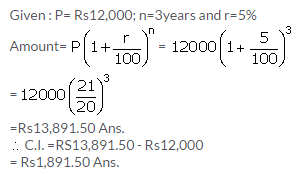Solution 2: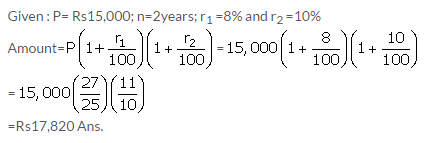Solution 3:Solution 4: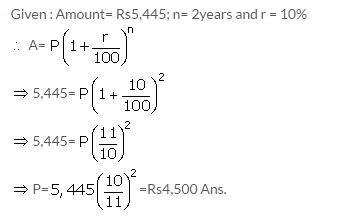Solution 5: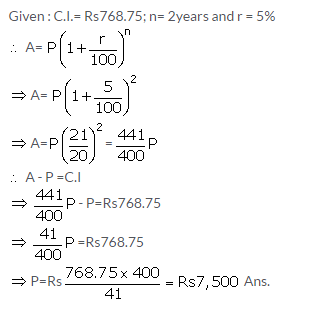Solution 6: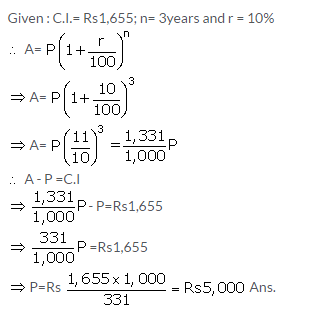Solution 7: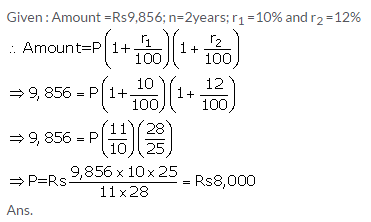Solution 8: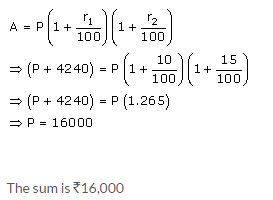Solution 9:At 5% per annum the sum of Rs.6,000 amounts to Rs.6,615 in 2 years when the interest is compounded annually.

Solution 10:
Let Rs.x and Rs.y be the money invested by Pramod and Rohit respectively such that they will get the same sum on attaining the age of 25 years.
Pramod will attain the age of 25 years after 25 – 16 = 9 years
Rohit will attain the age of 25 years after 25 -18 = 7 yearsPramod and Rohit should invest in 400:441 ratio respectively such that they will get the same sum on attaining the age of 25 years.

Solution 11: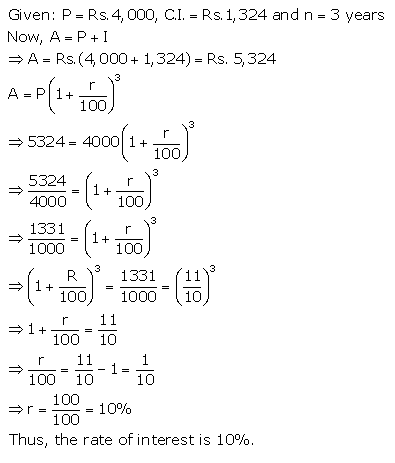Solution 12: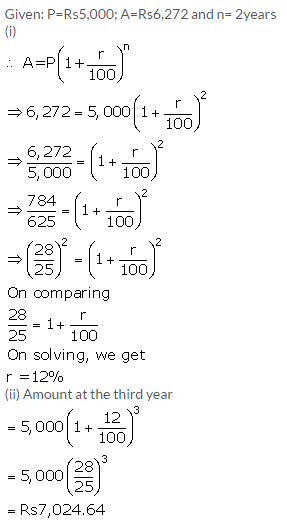Solution 13: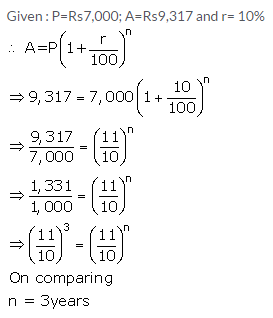Solution 14:Solution 15: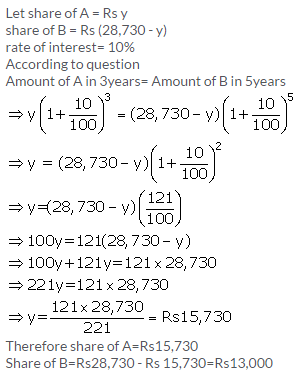Solution 16: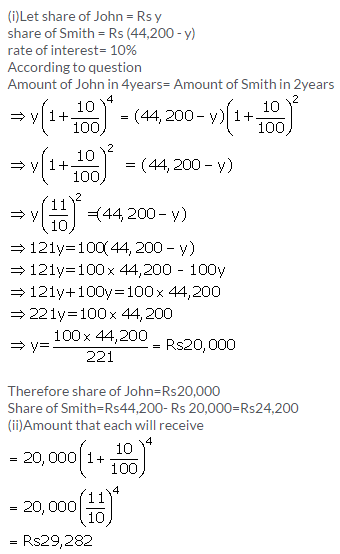Solution 17:Solution 18:Exercise 3(B)

Solution 1: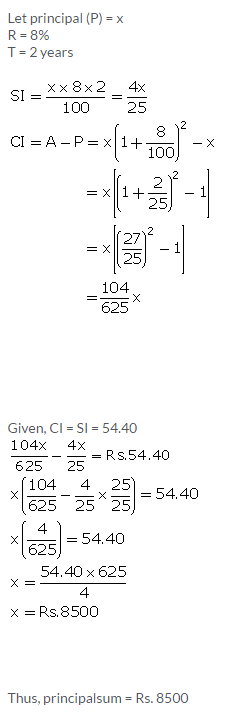Solution 2: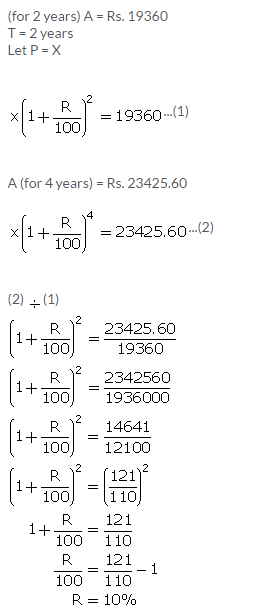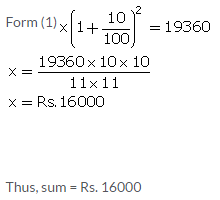Solution 3: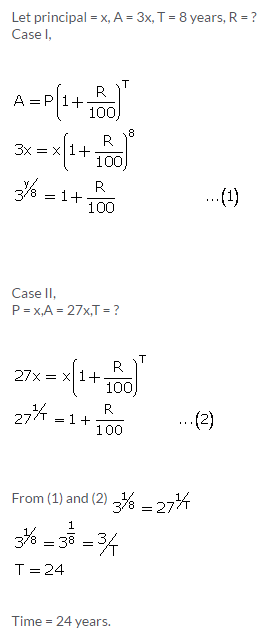Solution 4:Solution 5: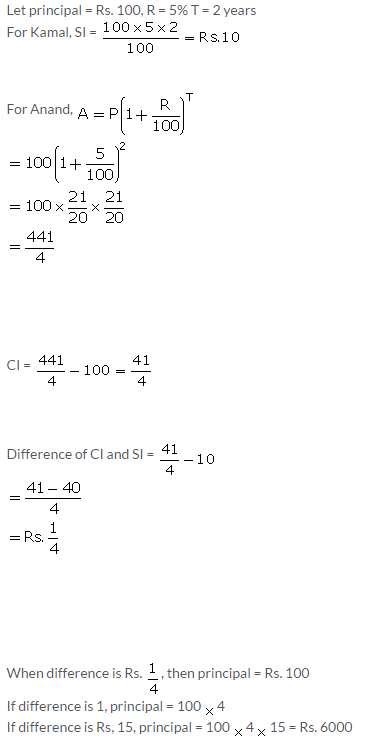Solution 6: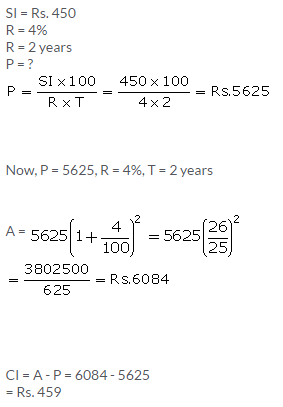Solution 7: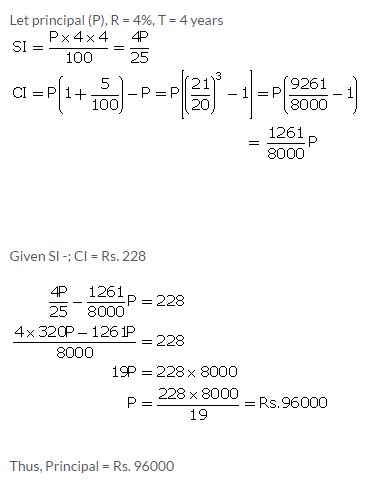Solution 8: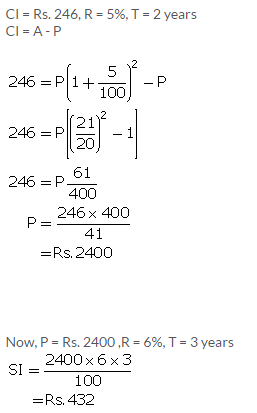Solution 9: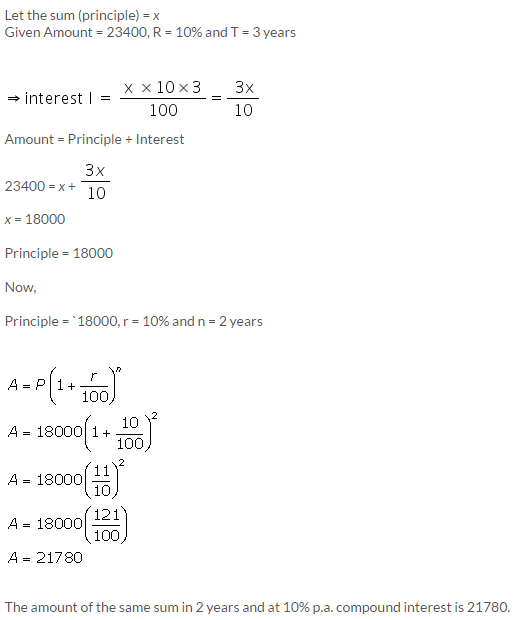Solution 10:Exercise 3(C)

Solution 1: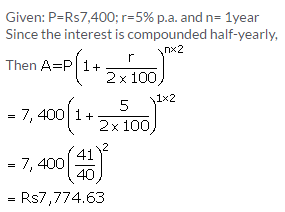Solution 2: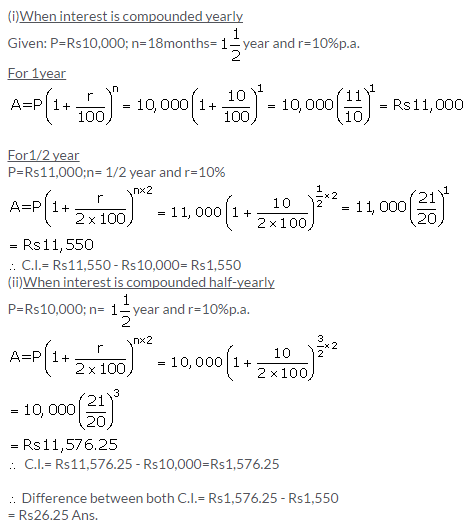Solution 3: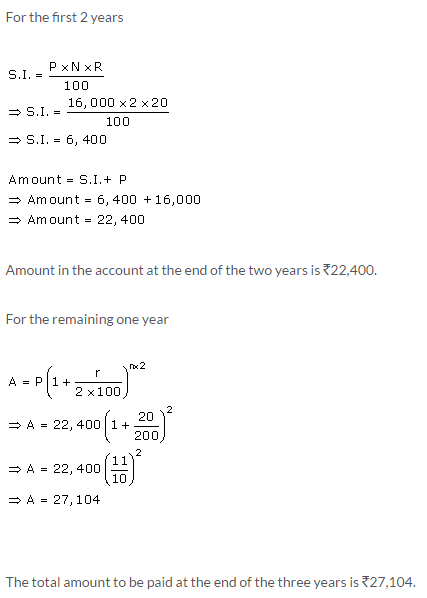Solution 4:Solution 5:Solution 6: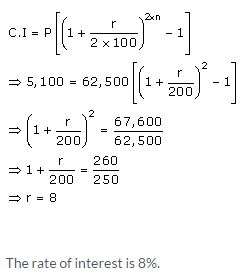Solution 7:Solution 8: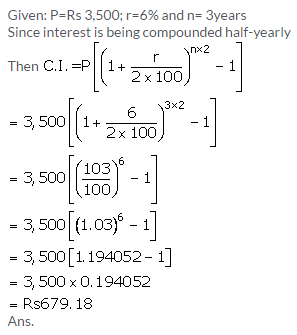Solution 9:Solution 10: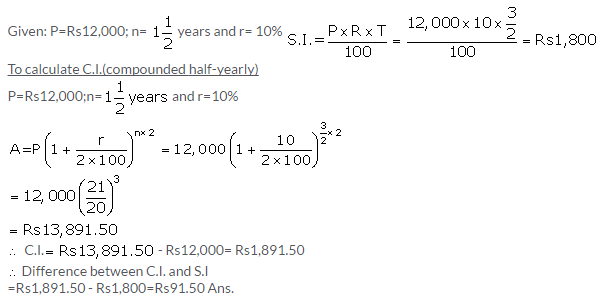Exercise 3(D)

Solution 1: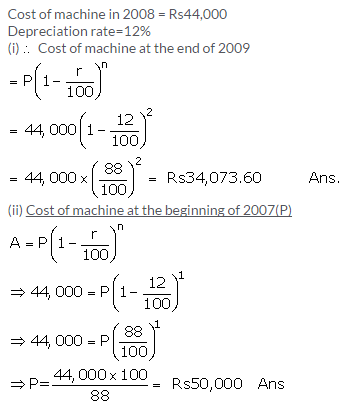Solution 2: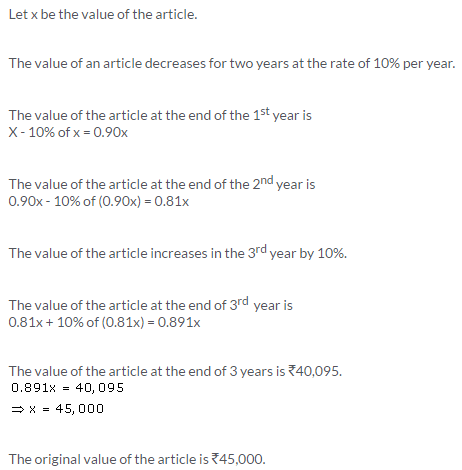Solution 3: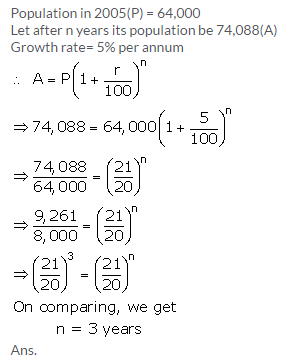Solution 4: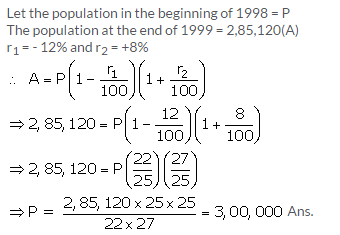Solution 5:Solution 6: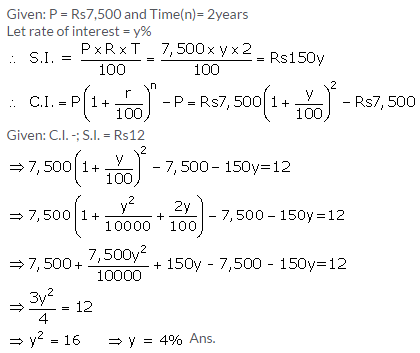Solution 7:Solution 8: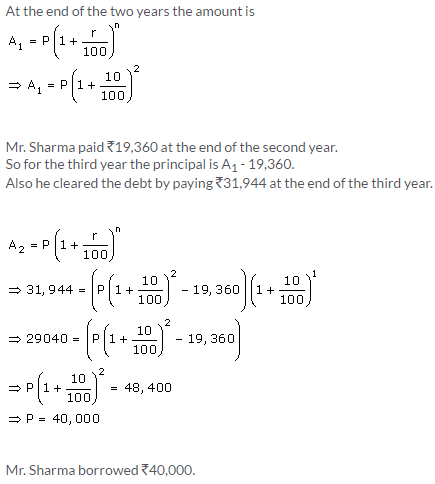Solution 9:Solution 10:Exercise 3(E)

Solution 1:Solution 2: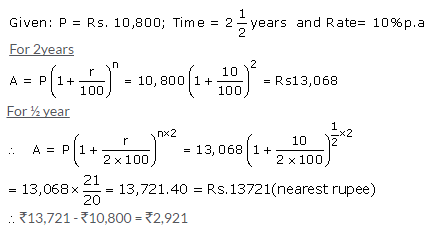Solution 3: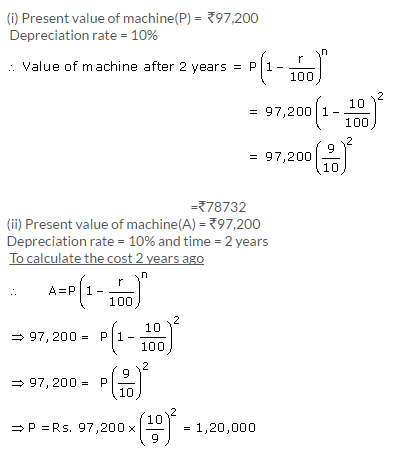Solution 4:Solution 5: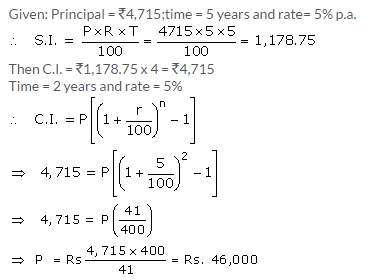Solution 6:Solution 7: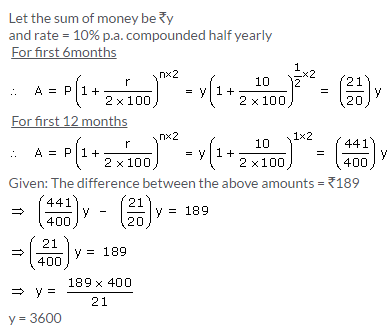Solution 8: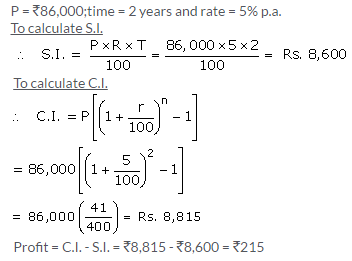Solution 9:Solution 10:More Resources for Selina Concise Class 9 ICSE Solutions